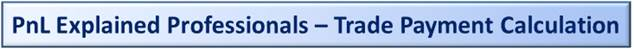Main Page by Topic

Thoughts and considerations for the design of trade payments how they are calculated.

Outline

1) What Is This?

We are talking about payments on trades, so, for example, for a fixed for float commodity swap that is one month long, you’ll have two ‘payments’, really one side a payment and one side a receipt.

Looks like this.  This assumes a volume of 11,000 units (e.g., BBL or GAL or MT or MMBTU) and a fixed price of \$51/unit and assume that market prices are currently \$52/unit.

 # Quantity Price Payment Side Type 1 -11,000 units \$51/unit \$(561,000) Fixed 2 11,000 units \$52/unit \$572,000 Index/Floating

Notes:

1.1) There are two rows, i.e., #1 and #2, to represent two ‘sides’ of the trade.  We are paying the fixed price, i.e., so paying \$561,000, so we show it as a negative.

1.2) We are receiving an estimated \$572,000, though this will change as market prices move up and down until the ‘fixing date’ of the payment.

1.3) The reality is that both payments would likely be netted (covered by a legal netting agreement), such the really only one side would pay.  If market prices stay as they are, i.e., remain at \$52/unit, then the counterparty of the trade would pay us \$11,000, which is the sum of the two payments.

2) Why Is The Important?

You’ll notice in the above example, the formula for the payment is just price times quantity.  Payment formulas can vary based on the trade type and commodity.

The problem is, CTRM system designers will often build into their systems very limited designs, assuming, for example, that payments will always be based on the simple price * quantity.

The reason why this is important, and the reason for this blog post, is that CTRM designers will often hard code rules about payment formulas by trade type, which is bad.

An interest rate swap or a loan, for example, will typically have a payment that is calculated like this:

Notional amount * interest rate * time in years = payment.

2.1) The notional amount would be something like \$300,000.

2.2) The interest rate would be something like 2.45%

2.3) And time in years would be like, 0.5 for 6 months.  The correct value of the ‘time in years’ will depend on Day Count Convention.  See:

https://en.wikipedia.org/wiki/Day_count_convention

For example, for a day count of ‘30/360’, 6 months will always be 0.5 of a year.  For a day count of Actual/360, the number of days in 6 months could be, for example, 182, which divided by 360 is 0.5055555.   So the formula remains notional * interest rate * time in years and it is just that the ‘time and years’ that will change.

For precious metals, e.g., gold, silver, platinum, palladium, they will also sometimes have payments based on an interest rate.  Two parties may agree for a fixed for float swap based on the exchange-traded futures price as of, for example, December.  Alternately, they may do a swap based on the ‘GOFO’ rate (the gold forward interest rate). See:

Because some systems just assume that you can only have a ‘quantity times price’ formula for commodities, if you want to trade gold based on interest rates, they will often introduce an unpleasant workaround of modelling gold as if it were a currency.  Because the system design only allows something setup as a ‘currency’ to be used with interest rate payment formulas.

Often then, firms need to setup gold as both a commodity and a currency, which can lead to different and inconsistent results even in the same system, such as how you keep track of your inventory of gold when pretending/modelling it as a currency while tracking other inventory of gold modelled as a commodity.

3) Design Considerations for CTRM

This post argues a better approach would be to treat all of the payments for any sort of trade in the system more generically.  For any trade, allow any kind of payment calculation.  In other words, on each trade, have a field where users can select the payment calculation type, e.g., ‘price*quantity’ or ‘interest rate based’.

If a user selects ‘interest rate based’ for a given payment, that would then open up / make available other fields that are needed, such as ‘Day Count Convention’.

Other types of payment calculations include:

3.1) For options.  E.g., for a call option, the payment formula is the maximum of two items, zero (i.e., \$0) or ‘market price minus strike price times quantity’.

3.2) Tiered pricing.  This would be applicable for physical deals where the physical volume delivered could be in a range.  E.g., you might have the first 8000 units at a fixed price of \$3/unit and then the next 4000 units, so 8001 to 12000 at an index price based off the NYMEX natural gas last day settle price plus an spread of \$0.05.

It would be expected to have default values, so users don’t need to worry about the specifics every time they book a trade.  And the takeaway/lesson is for a new system to design, right from the beginning, a very generic approach to payment calculations, such that a common model/design can work for all commodities, physical and financial, interest rate based and quantity * price based.

Introduction to CTRM

Click on this link for a great introduction to CTRM software: Introduction to CTRM Software